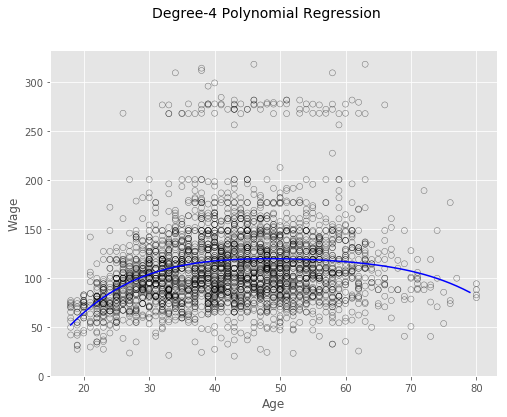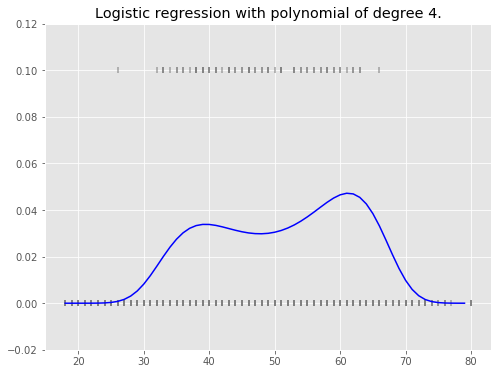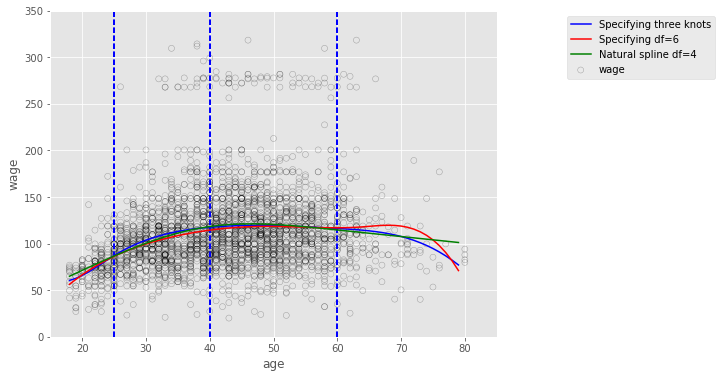# Chapter 7 - Moving Beyond Linearity¶

### Imports and Configurations¶

In :
# Standard imports
import warnings

from rpy2.robjects.packages import importr
from rpy2.robjects.packages import data as rdata
from rpy2.robjects import pandas2ri

# Math and data processing
import numpy as np
import scipy as sp
import pandas as pd

# StatsModels
import statsmodels.api as sm
import statsmodels.formula.api as smf
from statsmodels.stats.anova import anova_lm
from patsy import dmatrix

# scikit-learn
from sklearn.preprocessing import PolynomialFeatures

# Visulization
from IPython.display import display
import matplotlib as mpl
import matplotlib.pyplot as plt
%matplotlib inline
mpl.style.use('ggplot')


Load wage data set from R ISLR library.

In :
# wage dataset is in R ISLR package
islr = importr('ISLR')
wage_rdf = rdata(islr).fetch('Wage')['Wage']
wage = pandas2ri.ri2py(wage_rdf)

year age sex maritl race education region jobclass health health_ins logwage wage
231655 2006 18 1. Male 1. Never Married 1. White 1. < HS Grad 2. Middle Atlantic 1. Industrial 1. <=Good 2. No 4.318063 75.043154
86582 2004 24 1. Male 1. Never Married 1. White 4. College Grad 2. Middle Atlantic 2. Information 2. >=Very Good 2. No 4.255273 70.476020
161300 2003 45 1. Male 2. Married 1. White 3. Some College 2. Middle Atlantic 1. Industrial 1. <=Good 1. Yes 4.875061 130.982177
155159 2003 43 1. Male 2. Married 3. Asian 4. College Grad 2. Middle Atlantic 2. Information 2. >=Very Good 1. Yes 5.041393 154.685293
11443 2005 50 1. Male 4. Divorced 1. White 2. HS Grad 2. Middle Atlantic 2. Information 1. <=Good 1. Yes 4.318063 75.043154
In :
wage.info()

<class 'pandas.core.frame.DataFrame'>
Int64Index: 3000 entries, 231655 to 453557
Data columns (total 12 columns):
year          3000 non-null int32
age           3000 non-null int32
sex           3000 non-null object
maritl        3000 non-null object
race          3000 non-null object
education     3000 non-null object
region        3000 non-null object
jobclass      3000 non-null object
health        3000 non-null object
health_ins    3000 non-null object
logwage       3000 non-null float64
wage          3000 non-null float64
dtypes: float64(2), int32(2), object(8)
memory usage: 281.2+ KB


Utility function definition.

In :
def ortho_poly_fit(x, degree = 1):
'''
Convert data into orthogonal basis for polynomial regression by QR decomposition.
Ref: http://davmre.github.io/python/2013/12/15/orthogonal_poly
'''
n = degree + 1
x = np.asarray(x).flatten()
if(degree >= len(np.unique(x))):
stop("'degree' must be less than number of unique points")
xbar = np.mean(x)
x = x - xbar
X = np.fliplr(np.vander(x, n))
q,r = np.linalg.qr(X)

z = np.diag(np.diag(r))
raw = np.dot(q, z)

norm2 = np.sum(raw**2, axis=0)
alpha = (np.sum((raw**2)*np.reshape(x,(-1,1)), axis=0)/norm2 + xbar)[:degree]
Z = raw / np.sqrt(norm2)
return Z, norm2, alpha


### Lab 7.8.1 Polynomial Regression and Step Functions¶

Polynomial regression of degree 4 on orthogonalized X.

In :
# Polynomial regression of degree 4 on orthogonalized X. Refer to chapter 3 notebook.
X4_ortho = ortho_poly_fit(wage[['age']], degree=4)
X4_ortho[:,0]=1  # Replace constant column with 1s for Intercept estimation.
poly4_ortho = sm.GLS(wage['wage'], X4_ortho).fit()
print(poly4_ortho.summary())

                            GLS Regression Results
==============================================================================
Dep. Variable:                   wage   R-squared:                       0.086
Method:                 Least Squares   F-statistic:                     70.69
Date:                Tue, 21 Mar 2017   Prob (F-statistic):           2.77e-57
Time:                        18:05:48   Log-Likelihood:                -15315.
No. Observations:                3000   AIC:                         3.064e+04
Df Residuals:                    2995   BIC:                         3.067e+04
Df Model:                           4
Covariance Type:            nonrobust
==============================================================================
coef    std err          t      P>|t|      [95.0% Conf. Int.]
------------------------------------------------------------------------------
const        111.7036      0.729    153.283      0.000       110.275   113.132
x1           447.0679     39.915     11.201      0.000       368.805   525.331
x2          -478.3158     39.915    -11.983      0.000      -556.579  -400.053
x3           125.5217     39.915      3.145      0.002        47.259   203.785
x4           -77.9112     39.915     -1.952      0.051      -156.174     0.352
==============================================================================
Omnibus:                     1097.594   Durbin-Watson:                   1.960
Prob(Omnibus):                  0.000   Jarque-Bera (JB):             4965.521
Skew:                           1.722   Prob(JB):                         0.00
Kurtosis:                       8.279   Cond. No.                         54.8
==============================================================================

Warnings:
 Standard Errors assume that the covariance matrix of the errors is correctly specified.


Polynomial regression of degree 4 on raw X without orthogonalization.

In :
# Polynomial regression of degree 4 on raw X without orthogonalization.
X4 = PolynomialFeatures(degree=4).fit_transform(wage[['age']])
poly4 = sm.GLS(wage['wage'], X4).fit()
print(poly4.summary())

                            GLS Regression Results
==============================================================================
Dep. Variable:                   wage   R-squared:                       0.086
Method:                 Least Squares   F-statistic:                     70.69
Date:                Tue, 21 Mar 2017   Prob (F-statistic):           2.77e-57
Time:                        18:05:49   Log-Likelihood:                -15315.
No. Observations:                3000   AIC:                         3.064e+04
Df Residuals:                    2995   BIC:                         3.067e+04
Df Model:                           4
Covariance Type:            nonrobust
==============================================================================
coef    std err          t      P>|t|      [95.0% Conf. Int.]
------------------------------------------------------------------------------
const       -184.1542     60.040     -3.067      0.002      -301.879   -66.430
x1            21.2455      5.887      3.609      0.000         9.703    32.788
x2            -0.5639      0.206     -2.736      0.006        -0.968    -0.160
x3             0.0068      0.003      2.221      0.026         0.001     0.013
x4         -3.204e-05   1.64e-05     -1.952      0.051     -6.42e-05  1.45e-07
==============================================================================
Omnibus:                     1097.594   Durbin-Watson:                   1.960
Prob(Omnibus):                  0.000   Jarque-Bera (JB):             4965.521
Skew:                           1.722   Prob(JB):                         0.00
Kurtosis:                       8.279   Cond. No.                     5.67e+08
==============================================================================

Warnings:
 Standard Errors assume that the covariance matrix of the errors is correctly specified.
 The condition number is large, 5.67e+08. This might indicate that there are
strong multicollinearity or other numerical problems.


Predict over a grid of age.

In :
# Generate a sequence of age values spanning the range
age_grid = np.arange(wage.age.min(), wage.age.max()).reshape(-1,1)

# Generate test data
X_test = PolynomialFeatures(4).fit_transform(age_grid)

# Predict the value of the generated ages
y_pred = poly4.predict(X_test)

# Plot
fig, ax = plt.subplots(figsize=(8,6))
fig.suptitle('Degree-4 Polynomial Regression', fontsize=14)

# Scatter plot with polynomial regression line
plt.scatter(wage.age, wage.wage, facecolor='None', edgecolor='k', alpha=0.5)
plt.plot(age_grid, y_pred, color = 'b')
ax.set_ylim(ymin=0)
plt.xlabel('Age')
plt.ylabel('Wage')
plt.show()ANOVA on polynomial regression of degree from 1 to 5.

In :
# Polynomial regression of degree 1,2,3,5
X1 = PolynomialFeatures(degree=1).fit_transform(wage[['age']])
X2 = PolynomialFeatures(degree=2).fit_transform(wage[['age']])
X3 = PolynomialFeatures(degree=3).fit_transform(wage[['age']])
X5 = PolynomialFeatures(degree=5).fit_transform(wage[['age']])
poly1 = sm.GLS(wage['wage'], X1).fit()
poly2 = sm.GLS(wage['wage'], X2).fit()
poly3 = sm.GLS(wage['wage'], X3).fit()
poly5 = sm.GLS(wage['wage'], X5).fit()
# ANOVA, as in chpater 3 notebook
with warnings.catch_warnings():
warnings.filterwarnings("ignore")  ## Supress warnings
display(anova_lm(poly1, poly2, poly3, poly4, poly5))

df_resid ssr df_diff ss_diff F Pr(>F)
0 2998.0 5.022216e+06 0.0 NaN NaN NaN
1 2997.0 4.793430e+06 1.0 228786.010128 143.593107 2.363850e-32
2 2996.0 4.777674e+06 1.0 15755.693664 9.888756 1.679202e-03
3 2995.0 4.771604e+06 1.0 6070.152124 3.809813 5.104620e-02
4 2994.0 4.770322e+06 1.0 1282.563017 0.804976 3.696820e-01

The p-value comparing the cubic and degree-4 polynomials, Model 3 and Model 4, is approximately 5% while the degree-5 polynomial Model 5 seems unnecessary because its p-value is 0.37. Hence, either a cubic or a quartic polynomial appear to provide a reasonable fit to the data, but lower- or higher-order models are not justified.

In :
# Polynomial regression of degree 5 on orthogonalized X. Refer to chapter 3 notebook.
X5_ortho = ortho_poly_fit(wage[['age']], degree=5)
X5_ortho[:,0]=1  # Replace constant column with 1s for Intercept estimation.
poly5_ortho = sm.GLS(wage['wage'], X5_ortho).fit()
print(poly5_ortho.summary())

                            GLS Regression Results
==============================================================================
Dep. Variable:                   wage   R-squared:                       0.087
Method:                 Least Squares   F-statistic:                     56.71
Date:                Tue, 21 Mar 2017   Prob (F-statistic):           1.67e-56
Time:                        18:05:49   Log-Likelihood:                -15314.
No. Observations:                3000   AIC:                         3.064e+04
Df Residuals:                    2994   BIC:                         3.068e+04
Df Model:                           5
Covariance Type:            nonrobust
==============================================================================
coef    std err          t      P>|t|      [95.0% Conf. Int.]
------------------------------------------------------------------------------
const        111.7036      0.729    153.278      0.000       110.275   113.133
x1           447.0679     39.916     11.200      0.000       368.802   525.334
x2          -478.3158     39.916    -11.983      0.000      -556.582  -400.050
x3           125.5217     39.916      3.145      0.002        47.256   203.787
x4           -77.9112     39.916     -1.952      0.051      -156.177     0.355
x5           -35.8129     39.916     -0.897      0.370      -114.079    42.453
==============================================================================
Omnibus:                     1094.840   Durbin-Watson:                   1.961
Prob(Omnibus):                  0.000   Jarque-Bera (JB):             4940.229
Skew:                           1.718   Prob(JB):                         0.00
Kurtosis:                       8.265   Cond. No.                         54.8
==============================================================================

Warnings:
 Standard Errors assume that the covariance matrix of the errors is correctly specified.


Notice that the p-values are the same, and in fact the square of the t-statistics are equal to the F-statistics from the anova_lm() function.

Classification: wage > \$250,000 per year?

In :
# Create binary qualitative response
y_clf = (wage.wage > 250).map({False:0, True:1})
# Logistic regression
logreg = sm.GLM(y_clf, X4, family=sm.families.Binomial()).fit()
print(logreg.summary())

                 Generalized Linear Model Regression Results
==============================================================================
Dep. Variable:                   wage   No. Observations:                 3000
Model:                            GLM   Df Residuals:                     2995
Model Family:                Binomial   Df Model:                            4
Method:                          IRLS   Log-Likelihood:                -350.61
Date:                Tue, 21 Mar 2017   Deviance:                       701.22
Time:                        18:05:49   Pearson chi2:                 3.70e+03
No. Iterations:                    12
==============================================================================
coef    std err          z      P>|z|      [95.0% Conf. Int.]
------------------------------------------------------------------------------
const       -109.5530     47.655     -2.299      0.022      -202.956   -16.150
x1             8.9950      4.187      2.148      0.032         0.789    17.201
x2            -0.2816      0.135     -2.081      0.037        -0.547    -0.016
x3             0.0039      0.002      2.022      0.043         0.000     0.008
x4         -1.949e-05   9.91e-06     -1.966      0.049     -3.89e-05 -6.41e-08
==============================================================================

In :
# Predict on age grid
y_pred_clf = logreg.predict(X_test)
fig, ax = plt.subplots(figsize=(8, 6))
plt.plot(age_grid, y_pred_clf, color='b')
plt.ylim(-0.02, 0.12)
# Rug plot showing the distribution of wage>250 in the training data.
# 'True' on the top, 'False' on the bottom.
ax.scatter(wage.age, y_clf/10, s=30, c='grey', marker='|', alpha=0.7)
plt.title("Logistic regression with polynomial of degree 4.")
plt.show()Step functions for piecewise-constant regression

In :
# Cut predictor data into intervals of a step function
wage_cut, bins = pd.cut(wage.age, 4, retbins=True, right=True)
wage_cut.value_counts(sort=False)

Out:
(17.938, 33.5]     750
(33.5, 49]        1399
(49, 64.5]         779
(64.5, 80]          72
Name: age, dtype: int64
In :
# Create dummies for predictor intervals
wage_step_dummies = pd.get_dummies(wage_cut, drop_first=True)  # The first interval is the base and dropped
wage_step_dummies = pd.DataFrame(sm.add_constant(wage_step_dummies.values), columns=['(Intercept)'] + list(wage_step_dummies.columns.values), index=wage_step_dummies.index)

Out:
(Intercept) (33.5, 49] (49, 64.5] (64.5, 80]
231655 1.0 0.0 0.0 0.0
86582 1.0 0.0 0.0 0.0
161300 1.0 1.0 0.0 0.0
155159 1.0 1.0 0.0 0.0
11443 1.0 0.0 1.0 0.0
376662 1.0 0.0 1.0 0.0
450601 1.0 1.0 0.0 0.0
377954 1.0 0.0 0.0 0.0
228963 1.0 1.0 0.0 0.0
81404 1.0 0.0 1.0 0.0
302778 1.0 1.0 0.0 0.0
305706 1.0 1.0 0.0 0.0
8690 1.0 1.0 0.0 0.0
153561 1.0 1.0 0.0 0.0
449654 1.0 0.0 1.0 0.0
447660 1.0 0.0 1.0 0.0
160191 1.0 1.0 0.0 0.0
230312 1.0 0.0 1.0 0.0
301585 1.0 0.0 1.0 0.0
153682 1.0 1.0 0.0 0.0
158226 1.0 1.0 0.0 0.0
11141 1.0 1.0 0.0 0.0
448410 1.0 0.0 0.0 1.0
305116 1.0 1.0 0.0 0.0
233002 1.0 1.0 0.0 0.0
In :
# Piecewise-constant regression as a step function
logreg_step = sm.GLM(wage.wage, wage_step_dummies).fit()
print(logreg_step.summary())

                 Generalized Linear Model Regression Results
==============================================================================
Dep. Variable:                   wage   No. Observations:                 3000
Model:                            GLM   Df Residuals:                     2996
Model Family:                Gaussian   Df Model:                            3
Method:                          IRLS   Log-Likelihood:                -15353.
Date:                Tue, 21 Mar 2017   Deviance:                   4.8957e+06
Time:                        18:05:49   Pearson chi2:                 4.90e+06
No. Iterations:                     4
===============================================================================
coef    std err          z      P>|z|      [95.0% Conf. Int.]
-------------------------------------------------------------------------------
(Intercept)    94.1584      1.476     63.790      0.000        91.265    97.051
(33.5, 49]     24.0535      1.829     13.148      0.000        20.468    27.639
(49, 64.5]     23.6646      2.068     11.443      0.000        19.611    27.718
(64.5, 80]      7.6406      4.987      1.532      0.126        -2.135    17.416
===============================================================================


### Lab 7.8.2 Splines¶

Cubic splines

In :
# Specifying 3 knots
transformed_3knots = dmatrix("bs(wage.age, knots=(25,40,60), degree=3, include_intercept=False)",
{"wage.age": wage.age}, return_type='dataframe')

# Build a regular linear model from the splines
spln_3knots = sm.GLM(wage.wage, transformed_3knots).fit()
pred_3knots = spln_3knots.predict(dmatrix("bs(age_grid, knots=(25,40,60), degree=3, include_intercept=False)",
{"age_grid": age_grid}, return_type='dataframe'))
spln_3knots.params

Out:
Intercept                                                                 60.493714
bs(wage.age, knots=(25, 40, 60), degree=3, include_intercept=False)     3.980500
bs(wage.age, knots=(25, 40, 60), degree=3, include_intercept=False)    44.630980
bs(wage.age, knots=(25, 40, 60), degree=3, include_intercept=False)    62.838788
bs(wage.age, knots=(25, 40, 60), degree=3, include_intercept=False)    55.990830
bs(wage.age, knots=(25, 40, 60), degree=3, include_intercept=False)    50.688098
bs(wage.age, knots=(25, 40, 60), degree=3, include_intercept=False)    16.606142
dtype: float64
In :
# Specifying 6 degrees of freedom
transformed_deg6 = dmatrix("bs(wage.age, df=6, include_intercept=False)",
{"wage.age": wage.age}, return_type='dataframe')
spln_deg6 = sm.GLM(wage.wage, transformed_deg6).fit()
pred_deg6 = spln_deg6.predict(dmatrix("bs(age_grid, df=6, degree=3, include_intercept=False)",
{"age_grid": age_grid}, return_type='dataframe'))
spln_deg6.params

Out:
Intercept                                         56.313841
bs(wage.age, df=6, include_intercept=False)    27.824002
bs(wage.age, df=6, include_intercept=False)    54.062546
bs(wage.age, df=6, include_intercept=False)    65.828391
bs(wage.age, df=6, include_intercept=False)    55.812734
bs(wage.age, df=6, include_intercept=False)    72.131473
bs(wage.age, df=6, include_intercept=False)    14.750876
dtype: float64

Natural splines

In :
# Specifying 4 degrees of freedom
transformed_deg4 = dmatrix("cr(wage.age, df=4)", {"wage.age": wage.age}, return_type='dataframe')
spln_deg4 = sm.GLM(wage.wage, transformed_deg4).fit()
pred_deg4 = spln_deg4.predict(dmatrix("cr(age_grid, df=4)", {"age_grid": age_grid}, return_type='dataframe'))
spln_deg4.params

Out:
Intercept                79.642095
cr(wage.age, df=4)   -14.667784
cr(wage.age, df=4)    36.811142
cr(wage.age, df=4)    35.934874
cr(wage.age, df=4)    21.563863
dtype: float64
In :
# Plot splines
fig, ax = plt.subplots(figsize=(8, 6))
plt.scatter(wage.age, wage.wage, facecolor='None', edgecolor='k', alpha=0.3)
plt.plot(age_grid, pred_3knots, color='b', label='Specifying three knots')
plt.plot(age_grid, pred_deg6, color='r', label='Specifying df=6')
plt.plot(age_grid, pred_deg4, color='g', label='Natural spline df=4')
[plt.vlines(i , 0, 350, linestyles='dashed', lw=2, colors='b') for i in [25,40,60]]
plt.legend(bbox_to_anchor=(1.5, 1.0))
plt.xlim(15,85)
plt.ylim(0,350)
plt.xlabel('age')
plt.ylabel('wage');